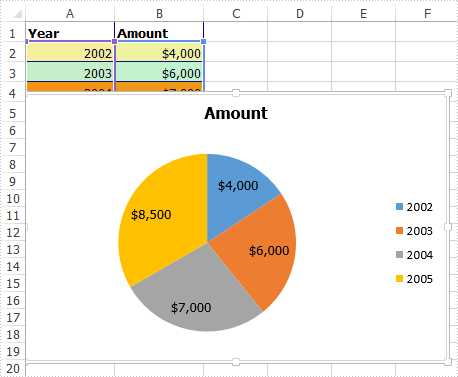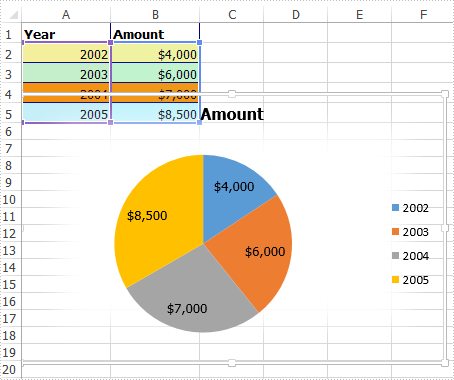# Apply Soft Edges effect to Excel Chart in C#

This article elaborates the steps to apply soft edges effect to an excel chart using Spire.XLS.

The example Excel file we used for demonstration:Detail steps:

Step 1: Instantiate a Workbook object and load the excel file.

```Workbook workbook = new Workbook();
```

Step 2: Get the first worksheet.

```Worksheet sheet = workbook.Worksheets;
```

Step 3: Get the chart.

```IChart chart = sheet.Charts;
```

Step 4: Specify the size of the soft edge. Value can be set from 0 to 100.

```chart.ChartArea.Shadow.SoftEdge = 10;
```

Step 5: Save the file.

```workbook.SaveToFile("Output.xlsx", ExcelVersion.Version2013);
```

Output:Full code:

```using Spire.Xls;
using Spire.Xls.Core;

namespace Soft_Edges_in_Excel_Chart
{
class Program
{
static void Main(string[] args)
{
//Instantiate a Workbook object
Workbook workbook = new Workbook();

//Get the first worksheet
Worksheet sheet = workbook.Worksheets;

//Get the chart
IChart chart = sheet.Charts;

//Specify the size of the soft edge. Value can be set from 0 to 100